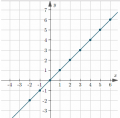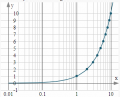# new equation of a function in semi-logarithm axis

Joined May 17, 2014
60
First, we plot the y=x, which is linear. now we want to plot this equation in semi-logarithm scale, in which the horizontal (x) is drawn logarithm. My question is what is the new equation of the y=x in the new plan. For more clarification please see these pictures.
the y=x isand the y=x in semi logarithm iswhat is the equation of above curve?

#### AlbertHall

Joined Jun 4, 2014
12,231
The equation is still y=x. It is only the graph scaling that has changed. The data is the same, only the display of the data has changed.

Joined May 17, 2014
60
Thank you. But, I want to know what is the new equation. For example in the new curve, I would like to calculate the slope of the curve in (1,1) point.

#### AlbertHall

Joined Jun 4, 2014
12,231
If you read the values from the scales on the graph the slope has not changed. It is still 1.
To calculate the slope at (2,2) we get the rise and run between the points either side of that point - (1,1) and (3,3). Rise=2 and run=2, so rise/run=1.

Only if you read values from the X-axis using some imposed linear scale - perhaps measuring the X values from the graph using a ruler - do you get anything else. In that case the curve will seem to be an exponential.

Joined May 17, 2014
60
yes. My mean is the slope of the exponential curve.
Indeed, I have a problem with the phase of the filters like low pass filter in the bode diagram.

#### AlbertHall

Joined Jun 4, 2014
12,231
Then the equation of the line, and therefore the slope, depends on what scale you pretend the X-axis has.

Joined May 17, 2014
60
OK. I understand.
In the Erickson book, there is a low pass filter that its phase change from 0 to -90 degree. The low pass filter equation is G(jw)=1/(1+j(w/w0)), which w0 denotes the radian centre frequency of the filter in which the phase of the filter is -45 degree. The phase of the filter is -tang-1(w/w0).
However, there are two points that their slop is equal to the slop of filter phase in w0. In the book, these two points are e^(-pi/2)w0 and e^(pi/2)w0. I want to know how these points are calculated. I sent the pic for clarification.

#### AlbertHall

Joined Jun 4, 2014
12,231
That's a question for someone else I'm afraid.

Joined May 17, 2014
60

Joined May 17, 2014
60

#### MrAl

Joined Jun 17, 2014
10,064
First, we plot the y=x, which is linear. now we want to plot this equation in semi-logarithm scale, in which the horizontal (x) is drawn logarithm. My question is what is the new equation of the y=x in the new plan. For more clarification please see these pictures.
the y=x is
View attachment 136466

and the y=x in semi logarithm is
View attachment 136467
what is the equation of above curve?
Hi,

I am not 100 percent sure what you want here, but if you use a conformal mapping of the space you get this:
log(a)/log(10)+j*(a+atan2(0,a))/log(10)

and what this gives you is a complete solution where you interpret the real part as units on the x axis and the imaginary part as the y axis. This spaces the x axis 1,10,100, 1000 as 0, 1, 2 ,3 which is just a long way of saying it's the log base 10.
So for your straight line we for a=0.01 we get the point (-2,0) and for a=0.1 we get (-1,0.1) and for a=1 we get (0,1) and for a=10 we get (1,10), etc.

•Joined May 17, 2014
60
Hi,

I am not 100 percent sure what you want here, but if you use a conformal mapping of the space you get this:
log(a)/log(10)+j*(a+atan2(0,a))/log(10)

and what this gives you is a complete solution where you interpret the real part as units on the x axis and the imaginary part as the y axis. This spaces the x axis 1,10,100, 1000 as 0, 1, 2 ,3 which is just a long way of saying it's the log base 10.
So for your straight line we for a=0.01 we get the point (-2,0) and for a=0.1 we get (-1,0.1) and for a=1 we get (0,1) and for a=10 we get (1,10), etc.
Thank you. How you achieved this relation?
I am thankful if you see my another question in this link.